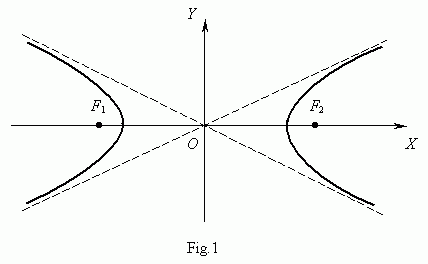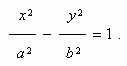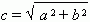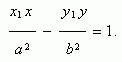Hyperbola

Hyperbola . Focuses . Equation of hyperbola. Focal length.

Real and imaginary axes of hyperbola. Eccentricity.

Asymptotes of hyperbola. Equation of tangent line to hyperbola.

Tangency condition of straight line and hyperbola.

A hyperbola ( Fig.1 ) is called a locus of points, a modulus of difference of distances from which to the two given points F 1 and F 2 , called focuses of hyperbola, is a constant value.An equation of hyperbola ( Fig.1 ) is :Here the origin of coordinates is a center of symmetry of hyperbola, and the coordinate axes are its axes of symmetry.

The segment F 1 F 2 = 2 ñ ,  whereis called a focal length . The segment AB = 2 a is called a real axis of hyperbola, the segment CD = 2 b is called an imaginary axis of hyperbola. The number e = c / a , e > 1 is called an eccentricity of hyperbola. The straight lines y = ± ( b / a ) x are called asymptotes of hyperbola .

Let Ð ( õ 1 , ó 1 )  be a point of hyperbola, then an equation of tangent line to hyperbola in this point is:A tangency condition of a straight line y = m x + k and a hyperbola õ 2 / a 2 ó 2 / b 2 = 1 :

k 2 = m 2 a 2 b 2 .